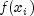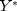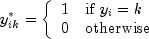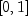JMSLTM Numerical Library 7.2.0
com.imsl.datamining

• All Implemented Interfaces:
Serializable, Cloneable

```public class GradientBoosting
extends PredictiveModel
implements Serializable, Cloneable```
Performs stochastic gradient boosting for a single response variable and multiple predictor variables.

The idea behind boosting is to combine the outputs of relatively weak classifiers or predictive models to achieve iteratively better and better accuracy in either regression problems (the response variable is continuous) or classification problems (the response variable has two or more discrete values). This class implements the stochastic gradient tree boosting algorithm of Friedman, 1999. A sequence of decision trees is fit to random samples of the training data, iteratively re-weighted to minimize a specified loss function. In each iteration, pseudo-residuals are calculated based on a random sample from the original training set and the gradient of the loss function evaluated at values generated in the previous iteration. New base predictors are fit to the pseudo-residuals, and then a new prediction function is selected to minimize the loss-function, completing one iteration. The number of iterations is a parameter for the algorithm.

Gradient boosting is an ensemble method, but instead of using independent trees, gradient boosting forms a sequence of trees, iteratively and judiciously re-weighted to minimize prediction errors. In particular, the decision tree at iteration m+1 is estimated on pseudo-residuals generated using the decision tree at step m. Hence, successive trees are dependent on previous trees. The algorithm in gradient boosting iterates for a fixed number of times and stops, rather than iterating until a convergence criteria is met. The number of iterations is therefore a parameter in the model. Using a randomly selected subset of the training data in each iteration has been shown to substantially improve efficiency and robustness. Thus, the method is called stochastic gradient boosting. For further discussion, see Hastie, et. al. (2008).

Example 1, Example 2, Example 3, Example 4, Serialized Form
• ### Nested Class Summary

Nested Classes
Modifier and Type Class and Description
`static class ` `GradientBoosting.LossFunctionType`
The loss function type as specified by the error measure.
• ### Nested classes/interfaces inherited from class com.imsl.datamining.PredictiveModel

`PredictiveModel.PredictiveModelException, PredictiveModel.StateChangeException, PredictiveModel.SumOfProbabilitiesNotOneException, PredictiveModel.VariableType`
• ### Constructor Summary

Constructors
Constructor and Description
```GradientBoosting(double[][] xy, int responseColumnIndex, PredictiveModel.VariableType[] varType)```
Constructs a `GradientBoosting` object for a single response variable and multiple predictor variables.
`GradientBoosting(PredictiveModel pm)`
Constructs a ` gradient boosting` object.
• ### Method Summary

Methods
Modifier and Type Method and Description
`void` `fitModel()`
Performs the gradient boosting on the training data.
`double[][]` `getClassFittedValues()`
Returns the fitted valuesfor a categorical response variable with two or more levels.
`double[][]` `getClassProbabilities()`
Returns the predicted probabilities on the training data for a categorical response variable.
`double[]` `getFittedValues()`
Returns the fitted valuesfor a continuous response variable after gradient boosting.
`int[]` `getIterationsArray()`
Returns the array of different values for the number of iterations.
`GradientBoosting.LossFunctionType` `getLossType()`
Returns the current loss function type.
`double` `getLossValue()`
Returns the loss function value.
`boolean` `getMissingTestYFlag()`
Returns the flag that sets whether the test data is missing the response variable data.
`double[][]` `getMultinomialResponse()`
Returns the multinomial representation of the response variable.
`int` `getNumberOfIterations()`
Returns the current setting for the number of iterations to use in the gradient boosting algorithm.
`double` `getSampleSizeProportion()`
Returns the current setting of the sample size proportion.
`double` `getShrinkageParameter()`
Returns the current shrinkage parameter.
`double[][]` `getTestClassFittedValues()`
Returns the fitted valuesfor a categorical response variable with two or more levels on the test data.
`double[][]` `getTestClassProbabilities()`
Returns the predicted probabilities on the test data for a categorical response variable.
`double[]` `getTestFittedValues()`
Returns the fitted valuesfor a continuous response variable after gradient boosting on the test data.
`double` `getTestLossValue()`
Returns the loss function value on the test data.
`double[]` `predict()`
Returns the predicted values on the training data.
`double[]` `predict(double[][] testData)`
Returns the predicted values on the input test data.
`double[]` ```predict(double[][] testData, double[] testDataWeights)```
Runs the gradient boosting on the training data and returns the predicted values on the weighted test data.
`void` `setIterationsArray(int[] iterationsArray)`
Sets the array of different numbers of iterations.
`void` `setLossFunctionType(GradientBoosting.LossFunctionType lossType)`
Sets the loss function type for the gradient boosting algorithm.
`void` `setMissingTestYFlag(boolean missingTestY)`
Sets the flag determining whether the test data is missing the response variable data.
`void` `setNumberOfIterations(int numberOfIterations)`
Sets the number of iterations.
`void` `setSampleSizeProportion(double sampleSizeProportion)`
Sets the sample size proportion.
`void` `setShrinkageParameter(double shrinkageParameter)`
Sets the value of the shrinkage parameter.
• ### Methods inherited from class com.imsl.datamining.PredictiveModel

`getClassCounts, getCostMatrix, getMaxNumberOfCategories, getNumberOfClasses, getNumberOfColumns, getNumberOfMissing, getNumberOfPredictors, getNumberOfRows, getNumberOfUniquePredictorValues, getPredictorIndexes, getPredictorTypes, getPrintLevel, getPriorProbabilities, getRandomObject, getResponseColumnIndex, getResponseVariableAverage, getResponseVariableMostFrequentClass, getResponseVariableType, getTotalWeight, getVariableType, getWeights, getXY, isMustFitModelFlag, isUserFixedNClasses, setClassCounts, setConfiguration, setCostMatrix, setFitModelFlag, setMaxNumberOfCategories, setNumberOfClasses, setPredictorIndex, setPredictorTypes, setPrintLevel, setPriorProbabilities, setRandomObject, setWeights`
• ### Methods inherited from class java.lang.Object

`clone, equals, finalize, getClass, hashCode, notify, notifyAll, toString, wait, wait, wait`
• ### Constructor Detail

```public GradientBoosting(double[][] xy,
int responseColumnIndex,
PredictiveModel.VariableType[] varType)```
Constructs a `GradientBoosting` object for a single response variable and multiple predictor variables.
Parameters:
`xy` - a `double` matrix containing the training data
`responseColumnIndex` - an `int`, the column index for the response variable
`varType` - a `PredictiveModel.VariableType` array containing the type of each variable

`public GradientBoosting(PredictiveModel pm)`
Constructs a ` gradient boosting` object.
Parameters:
`pm` - the `PredictiveModel` to serve as the base learner

Note: Currently only regression trees are supported as base learners.

• ### Method Detail

• #### fitModel

```public void fitModel()
throws PredictiveModel.PredictiveModelException```
Performs the gradient boosting on the training data.
Overrides:
`fitModel` in class `PredictiveModel`
Throws:
`PredictiveModel.PredictiveModelException` - An exception has occurred in the com.imsl.datamining.PredictiveModel. Superclass exceptions should be considered such as com.imsl.datamining.PredictiveModel.StateChangeException and com.imsl.datamining.PredictiveModel.SumOfProbabilitiesNotOneException.
• #### getClassFittedValues

`public double[][] getClassFittedValues()`
Returns the fitted valuesfor a categorical response variable with two or more levels.

The underlying loss function is the binomial or multinomial deviance.

Returns:
a `double` matrix containing the fitted values on the training data
• #### getClassProbabilities

`public double[][] getClassProbabilities()`
Returns the predicted probabilities on the training data for a categorical response variable.
Returns:
a `double` matrix containing the class probabilities fit on the training data. The i,k-th element of the matrix is the estimated probability that the observation at row index i belongs to the k+1-st class, where k=0,..., `nClasses`-1.
• #### getFittedValues

`public double[] getFittedValues()`
Returns the fitted valuesfor a continuous response variable after gradient boosting.
Returns:
a `double` array containing the fitted values on the training data
• #### getIterationsArray

`public int[] getIterationsArray()`
Returns the array of different values for the number of iterations.

Different values for the number of iterations can be set and used in cross validation. See `setIterationsArray(int[])`.

Returns:
an `int` array, containing the values for the number of iterations parameter
• #### getLossType

`public GradientBoosting.LossFunctionType getLossType()`
Returns the current loss function type.
Returns:
a `LossFunctionType`, the current setting of the loss function type
• #### getLossValue

`public double getLossValue()`
Returns the loss function value.
Returns:
a `double`, the loss function value
• #### getMissingTestYFlag

`public boolean getMissingTestYFlag()`
Returns the flag that sets whether the test data is missing the response variable data.
Returns:
a `boolean`, the flag setting whether the test data is missing the response variable values
• #### getMultinomialResponse

`public double[][] getMultinomialResponse()`
Returns the multinomial representation of the response variable.is a matrix with the element at i,k, where i=0,...,`nObservations`-1 and k=0,...,`nClasses`-1Note: This representation is not available if the response has only 2 classes (binomial).

Returns:
a `double` matrix containing the response in multinomial representation
• #### getNumberOfIterations

`public int getNumberOfIterations()`
Returns the current setting for the number of iterations to use in the gradient boosting algorithm.

Different values for the number of iterations can be set and used in cross validation. See `setIterationsArray(int[])`.

Returns:
an `int`, the current setting for the number of iterations
• #### getSampleSizeProportion

`public double getSampleSizeProportion()`
Returns the current setting of the sample size proportion.
Returns:
a `double`, the sample size proportion
• #### getShrinkageParameter

`public double getShrinkageParameter()`
Returns the current shrinkage parameter.
Returns:
a `double`, the value of shrinkage parameter
• #### getTestClassFittedValues

`public double[][] getTestClassFittedValues()`
Returns the fitted valuesfor a categorical response variable with two or more levels on the test data.

The underlying loss function is the binomial or multinomial deviance.

Returns:
a `double` matrix containing the fitted values on the test data
• #### getTestClassProbabilities

`public double[][] getTestClassProbabilities()`
Returns the predicted probabilities on the test data for a categorical response variable.
Returns:
a `double` matrix containing the class probabilities fit on the training data. The i,k element is the estimated probability that the i-th pattern belongs to the k-th target class, where k=0,...,`nClasses`-1.
• #### getTestFittedValues

`public double[] getTestFittedValues()`
Returns the fitted valuesfor a continuous response variable after gradient boosting on the test data.
Returns:
a `double` array containing the fitted values on the test data
• #### getTestLossValue

`public double getTestLossValue()`
Returns the loss function value on the test data.
Returns:
a `double`, the loss function value
• #### predict

```public double[] predict()
throws PredictiveModel.PredictiveModelException```
Returns the predicted values on the training data.
Specified by:
`predict` in class `PredictiveModel`
Returns:
a `double` array containing the predicted values on the training data, i.e., the fitted values
Throws:
`PredictiveModel.PredictiveModelException` - An exception has occurred in the com.imsl.datamining.PredictiveModel. Superclass exceptions should be considered such as com.imsl.datamining.PredictiveModel.StateChangeException and com.imsl.datamining.PredictiveModel.SumOfProbabilitiesNotOneException.
• #### predict

```public double[] predict(double[][] testData)
throws PredictiveModel.PredictiveModelException```
Returns the predicted values on the input test data.
Specified by:
`predict` in class `PredictiveModel`
Parameters:
`testData` - a `double` matrix containing test data

Note: `testData` must have the same number of columns and the columns must be in the same arrangement as `xy`.

Returns:
a `double` array containing the predicted values
Throws:
`PredictiveModel.PredictiveModelException` - An exception has occurred in the com.imsl.datamining.PredictiveModel. Superclass exceptions should be considered such as com.imsl.datamining.PredictiveModel.StateChangeException and com.imsl.datamining.PredictiveModel.SumOfProbabilitiesNotOneException.
• #### predict

```public double[] predict(double[][] testData,
double[] testDataWeights)
throws PredictiveModel.PredictiveModelException```
Runs the gradient boosting on the training data and returns the predicted values on the weighted test data.
Specified by:
`predict` in class `PredictiveModel`
Parameters:
`testData` - a `double` matrix containing test data

Note:`testData` must have the same number of columns and the columns must be in the same arrangement as `xy`.

`testDataWeights` - a `double` array containing weights for each row of `testData`
Returns:
a `double` array containing the predicted values
Throws:
`PredictiveModel.PredictiveModelException` - An exception has occurred in the com.imsl.datamining.PredictiveModel. Superclass exceptions should be considered such as com.imsl.datamining.PredictiveModel.StateChangeException and com.imsl.datamining.PredictiveModel.SumOfProbabilitiesNotOneException.
• #### setIterationsArray

`public void setIterationsArray(int[] iterationsArray)`
Sets the array of different numbers of iterations.

The algorithm in gradient boosting iterates for a fixed number of times and stops, rather than iterating until a convergence criteria is met. The number of iterations is therefore a parameter in the model. After setting the `iterationsArray` to two or more values, cross-validation can be used to help determine the best choice among the values. By default, `iterationsArray` contains the single value `{50}`.

Parameters:
`iterationsArray` - an `int` array containing the different numbers of iterations

Default: `iterationsArray` = {50}.

• #### setLossFunctionType

`public void setLossFunctionType(GradientBoosting.LossFunctionType lossType)`
Sets the loss function type for the gradient boosting algorithm.
Parameters:
`lossType` - a `LossFunctionType`, the desired loss function type

Default: `lossType`=`LossFunctionType.LEAST_SQUARES`

• #### setMissingTestYFlag

`public void setMissingTestYFlag(boolean missingTestY)`
Sets the flag determining whether the test data is missing the response variable data.
Parameters:
`missingTestY` - a `boolean`. When `true`, either the response variable data is all `Double.NaN` or will be treated as such.

Default: `missingTestY`=`false`

• #### setNumberOfIterations

`public void setNumberOfIterations(int numberOfIterations)`
Sets the number of iterations.
Parameters:
`numberOfIterations` - an `int`, the number of iterations

Default: `numberOfIterations` = 50. The `numberOfIterations` must be positive.

Note: This method resets `iterationsArray` to an array of length 1 containing `numberOfIterations`.

• #### setSampleSizeProportion

`public void setSampleSizeProportion(double sampleSizeProportion)`
Sets the sample size proportion.
Parameters:
`sampleSizeProportion` - a `double` in the intervalspecifying the desired sampling proportion

Default: `samplesSizeProportion` = 0.50. If `samplesSizeProportion` = 1.0, no sampling is performed.

• #### setShrinkageParameter

`public void setShrinkageParameter(double shrinkageParameter)`
Sets the value of the shrinkage parameter.
Parameters:
`shrinkageParameter` - a `double` in the intervalspecifying the shrinkage parameter

Default: `shrinkageParameter`=1.0 (no shrinkage)

JMSLTM Numerical Library 7.2.0